## The Works of Sonya Kovalevskaya

#### Extracts from article by Kimberly A. Meares http://home8.swipnet.se/~w-80790/Works/Kovalevs.htm

Possibly the most dazzling mathematical genius to surface among women during the past two centuries was the highly gifted Russian, Sofia Vasilyevna Kovalevskaya. She was born in Moscow on January 15, 1850 (3 January on the 19th century Russian Calendar), into a family of diverse backgrounds and talents. Sofia was destined to become a woman of great strengths veiled by great vulnerabilities, and the contributions she made to mathematics promise to be enduring ones.

Her doctoral dissertation, "On the Theory of Partial Differential Equations," dealt with a rather general system of differential equations of the first order in any number of variables. Weierstrass had already given an analogous structure for total equations; Sonya's paper extended this to partial differential equations. This is a remarkable contribution which was published in Crelle's Journal in 1875. These results are still of importance today and relevant in finding solutions to differential equations with initial conditions, this is known as the Cauchy problem. What follows is a modern version of what is commonly known as the

Cauchy-Kovalevskaya Theorem.

Let the partial differential equation be given in the form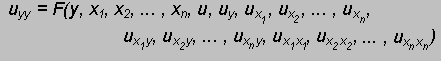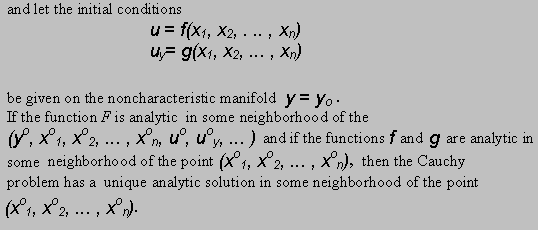The preceding statement seems equally applicable to hyperbolic, elliptic, and parabolic equations. However, we shall see that difficulties arise in formulating the Cauchy problem for nonhyperbolic equations. Consider, for instance, the Hadamard (1935) example. The problem consists of the elliptic equation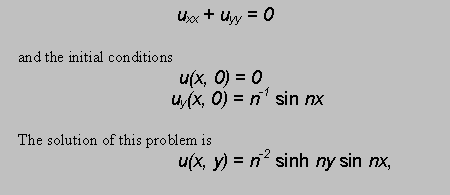which can be easily verified.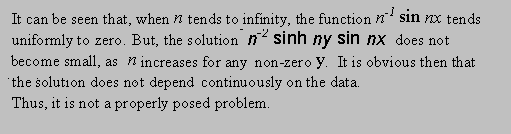In addition to existence and uniqueness, the question of continuous dependence of solution on the initial data arises in connection with the Cauchy-Kovalevskaya theorem. It is well known that any continuous function can accurately be approximated by polynomials. We can apply the Cauchy-Kovalevsky theorem with continuous data using polynomial approximations only if a small variation in the analytic data leads to a small change in the solution. For a parabolic equation, Kovalevskaya showed that the solution may not be analytic if the initial data are imposed on the wrong variable. Though there exist difficulties for equations of elliptic and parabolic type, this theorem is important for those of hyperbolic type, such as the wave equation.

Sonya also published "On the Reduction of a Definite Class of Abelian Integrals of the Third Range," again building on Weierstrass' earlier paper on the theory of Abelian integrals. This paper is of less importance but it consisted of a skilled series of manipulations which showed her complete command of Weierstrass's theory. Her other publications include a paper entitled "Supplementary Research and Observations on Laplace's Research on the Form of the Saturn Ring," and another paper, "On the Property of a System of Equations."

Some time in 1881, Weierstrass gave Kovalevskaya a mathematical project which indirectly lead her to achieve her most dazzling and important work. The particular problem he had in mind was the set of equations which Lame' had derived to describe the displacement of a particle in an elastic medium. Thus in mid 1881 Sonya settled down to work on a problem which was probably not of her own choosing, but soon after beginning, she found herself distracted by new insight into another problem of mathematical physics which had interested her many years earlier. This was the Euler equations which describe the motion of a rotating rigid body. The resulting paper took around 6 years to complete and was probably Kovalevskaya's greatest achievement. The mathematics involved go far beyond the scope of this paper, but I will attempt a brief description in modern notation:

The Euler equations are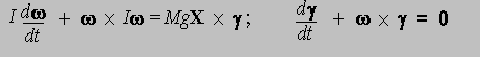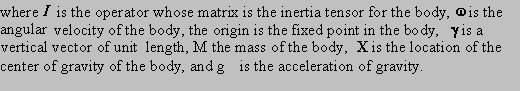These equations form a system of six first-order differential equations of great symmetry. Despite this great symmetry, however, the general solution had not been found. The integrals needed for the solution were at least hyperelliptic integrals, and probably even more complicated. The work of Jacobi (1849) had shown that the theory of theta functions might be profitably used to obtain a very explicit solution in a special case (X = 0) and had led Weierstrass to hope for solutions in more general cases using the theory developed for the Jacobi inversion problem. Evidently he had proposed this problem to Sonya while she was still a student.

Kovalevskaya's paper is assured of a permanent glory because it completes a program implicit in the works of Euler and Lagrange - to solve in an analytic manner the equations of motion. To do so requires cases in which there are enough algebraic integrals to permit a reduction to quadratures, and then a transformation of variables suitable for allowing an application of theta functions. Also, the modern day importance of Sonya's work on the dynamics of the top stem from her use of the study of movable poles to determine the integrability of the dynamical system. The location of the poles in the complex plane depends on the initial conditions for the system of differential equations, and it has only recently been recognized that her approach can apply to other systems. It was not even understood at that time why her methods worked, and it is quite notable that she was using complex analysis, a branch of mathematics that was still very much in its infancy. It is speculated that Sonya's work in this problem may have been inspired by Fuchs on the study of first order differential equations in the complex plane.

The high point of Sonya's career came on Christmas Eve of 1888, when she was presented the famous Prix Bordin of the French Academy of Sciences in recognition of her winning memoir On the Problem of the Rotation of a Solid Body about a Fixed Point. The rules of the competition for such prizes dictated that each entry be submitted anonymously. The author's name was sealed into an envelope bearing the same motto as that inscribed on the memoir, and the envelope was not to be opened until after the competing work won the prize. So when the jury of the Academy chose Sonya's entry, it was in utter ignorance that the winner was a women. The excellence of her entry was judged to be so exceptional that the value of the prize was increased from 3,000 francs to 5,000 francs "on account of the quite extraordinary service rendered to mathematical physics by this work." Incidentally, the motto on Sonya's prize-winning essay was "Say what you know, do what you must, come what may."

In the following year, the Stockholm Academy also honored Sonya with a prize of 1,500 kroner for two more works built on her original essay. In the same year, 1889 on the initiative of Chebyshev, Sonya was the first woman Corresponding Member of the Imperial Academy of Sciences. Her last published work was a short article Sur un théorème de M. Bruns in which she gave a new, simpler proof of Bruns' theorem on a property of the potential function of a homogeneous body. On February 10, 1891 at the height of her mathematical powers and reputation, Sonya Kovalevskaya died in Stockholm of influenza complicated by pneumonia.

Back to Selected Works page